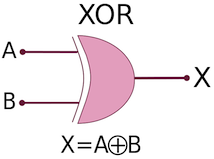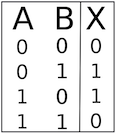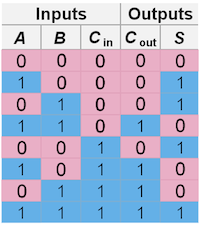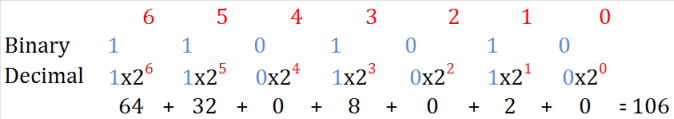# Boolean Logic Based Devices

## What is a logic gate?

A logic gate is an idealized or physical device implementing a Boolean function. In other terms it performs a logical operation on one or more logical inputs and produces a single logical output (From Wikipedia). Simply said: logic gates are the devices that perform the boolean logic operations.

AND Gate

For an AND gate, if the two inputs are equal to 1, the result equals 1.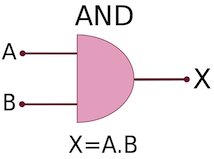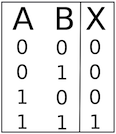OR Gate

For an OR gate, if one or the other (or both) of the inputs are equal to 1, the result equals 1.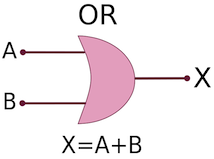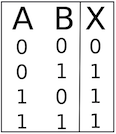XOR Gate

For a XOR gate, if one or the other (but not both of them) of the inputs are equal to 1, the result equals 1.